中文版 | EnglishSupport
 Technical Support FAQ After Service Sales NetworkTel:86 755 27308212 / 27307702 / 27308249 / 27307589
Fax:86 755 27307986
Web:www.szkemin.com
E-mail:qiuyangqi@szkemin.com, amanda.zhou@szkemin.com
Add:1-4F,NO.101, Building 20, New Development Zone ,East District,Baishixia,Fu Yong Street,Bao'an District,Shenzhen, Guangdong, China
Code:518103
Technical Support
The working principle of NTC negative temperature coefficient of resistance
Visit: Date:2013-11-29NTC is Negative Temperature Coefficient acronym, meaning is negative temperature coefficient, refers to the negative temperature coefficient large semiconductor materials or components, the so-called NTC thermistor is the negative temperature coefficient thermistor. It is based on the manganese, cobalt, nickel and copper and other metal oxide as the main material, made of ceramic manufacturing process. These metal oxide materials have semiconductor properties, because the way completely similar to the conductive germanium, silicon and other semiconductor materials. When the temperature is low, the oxide material carriers (electron and hole) the number of small, so its resistance value is higher; as the temperature increases, the number of carrier increases, so the resistance decreases. NTC thermistor at room temperature changes in the range of 10O~1000000 ohm, temperature coefficient -2%~-6.5%. NTC thermistor can be widely used in temperature measurement, temperature compensation, suppress the inrush current.

NTC negative temperature coefficient thermistor terminology

Zero power resistance value RT (Ω)

RT refers to the prescribed temperature T, caused by the resistance value variation of resistance with respect to measurement error in general can be ignored measured power regardless of measured values.

The relationship between resistance value and temperature change for:

RT = RN expB (1/T – 1/TN)

RT: at the temperature of T (K) NTC thermistor.
RN: in the rated temperature TN (K) NTC thermistor.
T: the specified temperature (K).
B: the material constants of NTC thermistor, also called thermal index.
Exp: with the natural number e as the base index (E = 2.71828...).

This relationship is the empirical formula, certain precision is only in a limited range of temperature rating of TN or the nominal resistance RN, because the function of material constants of B itself is temperature T.

Rated zero power resistance value R25 (Ω)

According to the national standard, rated zero power resistance is the resistance of NTC thermistor in the reference temperature of 25 ℃ measured value is R25, the resistance value is the nominal resistance values of NTC thermistor. The NTC thermistor much resistance, also refers to the value.

The material constants (thermal index) value of B (K)

The B value is defined as: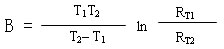RT1: temperature of T1 (K) zero power resistance value.
RT2: temperature of T2 (K) zero power resistance value.
T1, T2: two designated temperature (K).

The NTC thermistor used, generally in the range 2000K to 6000K B.

Temperature coefficient of zero power resistance (α T)

At a specified temperature, NTC thermistor zero dynamic changes in the relative power resistance value caused by the change of temperature and the ratio of the value of the.Alpha T: temperature of T (K) temperature coefficient of zero power resistance.
RT: temperature of T (K) zero power resistance value.
T: temperature (T).
B: material constants.

Dissipation factor (δ)

In the specified ambient temperature, NTC thermistor dissipation coefficient is the ratio of power and resistance changes in body temperature dissipation corresponding change.δ: NTC thermistor dissipation factor (mW/, K).
△ P: power consumption of NTC thermistor (mW).
△ T: NTC thermistor, power consumption of △ P, temperature change resistance corresponding body (K).

Thermal time constant (τ)

In the zero power condition, when the temperature changes, temperature of the thermistor changes before two temperature difference of 63.2% time, the thermal time constant and thermal capacity of NTC thermistor is proportional to, and the dissipation coefficient is inversely proportional to.Tau: thermal time constant (S).
Heat capacity C: of NTC thermistor.
Delta: dissipation coefficient NTC thermistor.

Rated power Pn

In the technology of the prescribed conditions, power thermistor long-term continuous work allows consumption. This power, resistance body temperature does not exceed the maximum working temperature.

The maximum working temperature of Tmax

In the technology of the prescribed conditions, the maximum temperature of the thermistor can allow long-term continuous working. That is: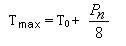The T0- environment temperature.

Measurement of power Pm

Thermistor at specified ambient temperature, resistance by measuring current heating induced resistance changes relative to the total error is negligible power consumption without timing.
General requirements for resistance change is greater than 0.1%, the measured power Pm for the time of: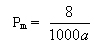Resistance temperature characteristic

The NTC thermistor temperature characteristics available type approximation:Type in:
RT: temperature T zero power resistance value.
A: coefficient associated with the physical properties of the materials and the geometric size of the resistor thermal.
The value of B:B.
T: temperature (K).
More accurate expressions for the: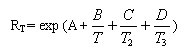Type in:

RT: zero power resistance at a temperature of T thermistor value.
T: is the absolute temperature value, K;
A, B, C, D: for specific constants.

NTC negative temperature coefficient thermistor R-T characteristics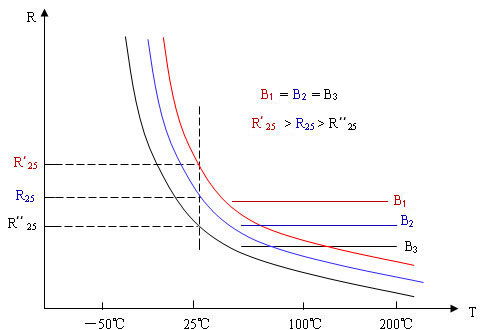B values are the same, the schematic diagram of R-T curves of different resistance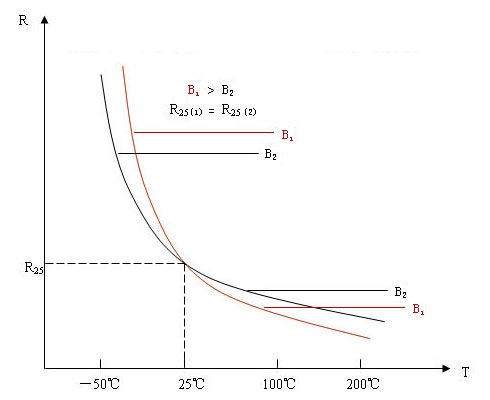The same resistance, the schematic diagram of NTC thermistor R-T curves of different B value

Temperature measurement, control with NTC thermistor

Appearance and structure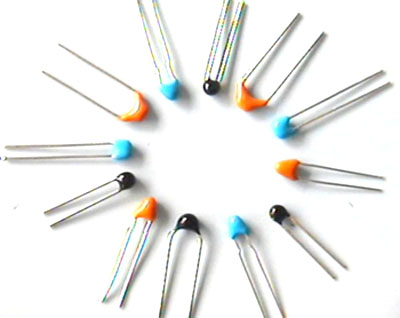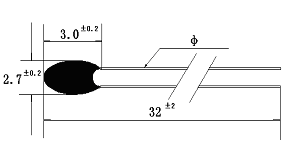Epoxy package series NTC thermistor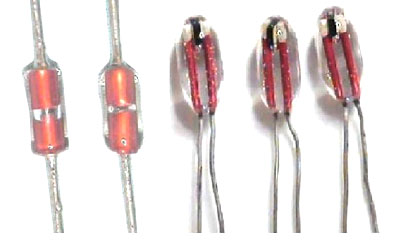Glass packaging series NTC thermistor

Application of the circuit principle diagram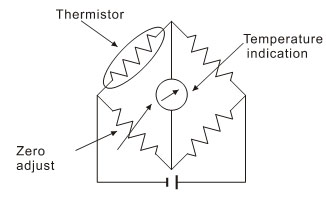Temperature measurement (Wheatstone bridge circuit)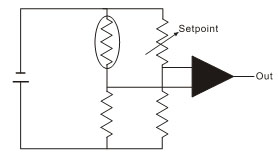Temperature control

Application design

• Electronic thermometer, electronic calendar, electronic clock and temperature display, electronic gifts;

• Cooling and heating equipment, heating constant temperature electric appliance;

• Automobile electronic temperature control circuit;

• The temperature sensor, temperature instrument;

• Medical electronic equipment, electronic toilet facilities;

• Mobile phone battery and charging appliance.

Model parameters

Epoxy package series NTC thermistor

 Model Nominal resistance values @25C (K Ω) The value of B (25/50C) (K) Rated power (MW) Dissipation coefficient (mw/C) Thermal time constant (S) Working temperature (C) MF52E-□ □ 3100 0.1~20 3100 50 2 In still air 7 In still air -55~+125 MF52E-□ □ 3270 0.2~20 3270 MF52E-□ □ 3380 0.5~50 3380 MF52E-□ □ 3470 0.5~50 3470 MF52E-□ □ 3600 1~100 3600 MF52E-□ □ 3950 5~100 3950 MF52E-□ □ 4000 5~100 4000 MF52E-□ □ 4050 5~200 4050 MF52E-□ □ 4150 10~250 4150 MF52E-□ □ 4300 20~1000 4300 MF52E-□ □ 4500 20~1000 4500

Note: 1 in the first box filled with nominal resistance, second box filling precision code. (F:± 1%G:± 2%H:± 3%J:± 5%)

The value of 2:B (25/50 ℃) error: for nominal resistance accuracy of ± 1% products of its corresponding B value error is ± 1%, the rest of the B error is ± 2%

Glass packaging series NTC thermistor

 Model Nominal resistance values @25C (K Ω) The value of B (25/50C) (K) Dissipation coefficient (mw/C) Thermal time constant (S) Working temperature (C) MF58-□ □ 3470 2 3470 2 In still air 20 In still air -55~+300 MF58-□ □ 3500 3 3500 MF58-□ □ 3500 5 3500 MF58-□ □ 3550 10 3550 MF58-□ □ 3600 15 3600 MF58-□ □ 3700 10 3700 MF58-□ □ 3850 20 3850 MF58-□ □ 3900 30 3900 MF58-□ □ 3950 47 3950 MF58-□ □ 3950 50 3950 MF58-□ □ 3990 100 3990 MF58-□ □ 4100 150 4100

Note: 1 in the first box filled with nominal resistance, second box filling precision code. (F:± 1%G:± 2%H:± 3%J:± 5%)

The value of 2:B (25/50 ℃) error: for nominal resistance accuracy of ± 1% products of its corresponding B value error is ± 1%, the rest of the B error is ± 2%

NTC is Negative Temperature Coefficient acronym, meaning is negative temperature coefficient, refers to the negative temperature coefficient large semiconductor materials or components, the so-called NTC thermistor is the negative temperature coefficient thermistor. It is based on the manganese, cobalt, nickel and copper and other metal oxide as the main material, made of ceramic manufacturing process. These metal oxide materials have semiconductor properties, because the way completely similar to the conductive germanium, silicon and other semiconductor materials. When the temperature is low, the oxide material carriers (electron and hole) the number of small, so its resistance value is higher; as the temperature increases, the number of carrier increases, so the resistance decreases. NTC thermistor at room temperature changes in the range of 10O~1000000 ohm, temperature coefficient -2%~-6.5%. NTC thermistor can be widely used in temperature measurement, temperature compensation, suppress the inrush current.

NTC negative temperature coefficient thermistor terminology

Zero power resistance value RT (Ω)

RT refers to the prescribed temperature T, caused by the resistance value variation of resistance with respect to measurement error in general can be ignored measured power regardless of measured values.

The relationship between resistance value and temperature change for:

RT = RN expB (1/T – 1/TN)

RT: at the temperature of T (K) NTC thermistor.
RN: in the rated temperature TN (K) NTC thermistor.
T: the specified temperature (K).
B: the material constants of NTC thermistor, also called thermal index.
Exp: with the natural number e as the base index (E = 2.71828...).

This relationship is the empirical formula, certain precision is only in a limited range of temperature rating of TN or the nominal resistance RN, because the function of material constants of B itself is temperature T.

Rated zero power resistance value R25 (Ω)

According to the national standard, rated zero power resistance is the resistance of NTC thermistor in the reference temperature of 25 ℃ measured value is R25, the resistance value is the nominal resistance values of NTC thermistor. The NTC thermistor much resistance, also refers to the value.

The material constants (thermal index) value of B (K)

The B value is defined as:RT1: temperature of T1 (K) zero power resistance value.
RT2: temperature of T2 (K) zero power resistance value.
T1, T2: two designated temperature (K).

The NTC thermistor used, generally in the range 2000K to 6000K B.

Temperature coefficient of zero power resistance (α T)

At a specified temperature, NTC thermistor zero dynamic changes in the relative power resistance value caused by the change of temperature and the ratio of the value of the.Alpha T: temperature of T (K) temperature coefficient of zero power resistance.
RT: temperature of T (K) zero power resistance value.
T: temperature (T).
B: material constants.

Dissipation factor (δ)

In the specified ambient temperature, NTC thermistor dissipation coefficient is the ratio of power and resistance changes in body temperature dissipation corresponding change.δ: NTC thermistor dissipation factor (mW/, K).
△ P: power consumption of NTC thermistor (mW).
△ T: NTC thermistor, power consumption of △ P, temperature change resistance corresponding body (K).

Thermal time constant (τ)

In the zero power condition, when the temperature changes, temperature of the thermistor changes before two temperature difference of 63.2% time, the thermal time constant and thermal capacity of NTC thermistor is proportional to, and the dissipation coefficient is inversely proportional to.Tau: thermal time constant (S).
Heat capacity C: of NTC thermistor.
Delta: dissipation coefficient NTC thermistor.

Rated power Pn

In the technology of the prescribed conditions, power thermistor long-term continuous work allows consumption. This power, resistance body temperature does not exceed the maximum working temperature.

The maximum working temperature of Tmax

In the technology of the prescribed conditions, the maximum temperature of the thermistor can allow long-term continuous working. That is:The T0- environment temperature.

Measurement of power Pm

Thermistor at specified ambient temperature, resistance by measuring current heating induced resistance changes relative to the total error is negligible power consumption without timing.
General requirements for resistance change is greater than 0.1%, the measured power Pm for the time of:Resistance temperature characteristic

The NTC thermistor temperature characteristics available type approximation:Type in:
RT: temperature T zero power resistance value.
A: coefficient associated with the physical properties of the materials and the geometric size of the resistor thermal.
The value of B:B.
T: temperature (K).
More accurate expressions for the:Type in:

RT: zero power resistance at a temperature of T thermistor value.
T: is the absolute temperature value, K;
A, B, C, D: for specific constants.

NTC negative temperature coefficient thermistor R-T characteristicsB values are the same, the schematic diagram of R-T curves of different resistanceThe same resistance, the schematic diagram of NTC thermistor R-T curves of different B value

Temperature measurement, control with NTC thermistor

Appearance and structureEpoxy package series NTC thermistorGlass packaging series NTC thermistor

Application of the circuit principle diagramTemperature measurement (Wheatstone bridge circuit)Temperature control

Application design

• Electronic thermometer, electronic calendar, electronic clock and temperature display, electronic gifts;

• Cooling and heating equipment, heating constant temperature electric appliance;

• Automobile electronic temperature control circuit;

• The temperature sensor, temperature instrument;

• Medical electronic equipment, electronic toilet facilities;

• Mobile phone battery and charging appliance.

Model parameters

Epoxy package series NTC thermistor

 Model Nominal resistance values @25C (K Ω) The value of B (25/50C) (K) Rated power (MW) Dissipation coefficient (mw/C) Thermal time constant (S) Working temperature (C) MF52E-□ □ 3100 0.1~20 3100 50 2 In still air 7 In still air -55~+125 MF52E-□ □ 3270 0.2~20 3270 MF52E-□ □ 3380 0.5~50 3380 MF52E-□ □ 3470 0.5~50 3470 MF52E-□ □ 3600 1~100 3600 MF52E-□ □ 3950 5~100 3950 MF52E-□ □ 4000 5~100 4000 MF52E-□ □ 4050 5~200 4050 MF52E-□ □ 4150 10~250 4150 MF52E-□ □ 4300 20~1000 4300 MF52E-□ □ 4500 20~1000 4500

Note: 1 in the first box filled with nominal resistance, second box filling precision code. (F:± 1%G:± 2%H:± 3%J:± 5%)

The value of 2:B (25/50 ℃) error: for nominal resistance accuracy of ± 1% products of its corresponding B value error is ± 1%, the rest of the B error is ± 2%

Glass packaging series NTC thermistor

 Model Nominal resistance values @25C (K Ω) The value of B (25/50C) (K) Dissipation coefficient (mw/C) Thermal time constant (S) Working temperature (C) MF58-□ □ 3470 2 3470 2 In still air 20 In still air -55~+300 MF58-□ □ 3500 3 3500 MF58-□ □ 3500 5 3500 MF58-□ □ 3550 10 3550 MF58-□ □ 3600 15 3600 MF58-□ □ 3700 10 3700 MF58-□ □ 3850 20 3850 MF58-□ □ 3900 30 3900 MF58-□ □ 3950 47 3950 MF58-□ □ 3950 50 3950 MF58-□ □ 3990 100 3990 MF58-□ □ 4100 150 4100

Note: 1 in the first box filled with nominal resistance, second box filling precision code. (F:± 1%G:± 2%H:± 3%J:± 5%)

The value of 2:B (25/50 ℃) error: for nominal resistance accuracy of ± 1% products of its corresponding B value error is ± 1%, the rest of the B error is ± 2%Copyright @ 2013 Shenzhen Kemin Sensor Co.,Ltd. AllRight Reserved
Tel:86 755 27308212/27307702/27308249/27307589 Fax:86 755 27307986